## Introduction to Sorting Techniques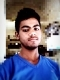Updated on 30-Jul-2019 22:30:23
Sorting refers to arranging data in a particular format. Sorting algorithm specifies the way to arrange data in a particular order. Most common orders are in numerical or lexicographical order. The importance of sorting lies in the fact that data searching can be optimized to a very high level, if data is stored in a sorted manner. Sorting is also used to represent data in more readable formats. In this Section We are going to cover − Bubble Sort Bucket Sort Comb Sort Counting Sort Cycle Sort Heap Sort Insertion Sort Merge Sort Pigeonhole Sort Quick Sort Radix Sort ... Read More

## Shell SortUpdated on 15-Jun-2020 14:58:31
The shell sorting technique is based on the insertion sort. In the insertion sort sometimes we need to shift large block to insert an item in the correct location. Using shell sort, we can avoid a large number of shifting. The sorting is done with a specific interval. After each pass, the interval is reduced to make the smaller interval.The complexity of the Shell Sort TechniqueTime Complexity: O(n log n) for best case, and for other cases, it depends on the gap sequence.Space Complexity: O(1)Input and OutputInput: The unsorted list: 23 56 97 21 35 689 854 12 47 66 ... Read More

## Selection Sort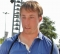Updated on 15-Jun-2020 15:02:02
In the selection sort technique, the list is divided into two parts. In one part all elements are sorted and in another part the items are unsorted. At first, we take the maximum or minimum data from the array. After getting the data (say minimum) we place it at the beginning of the list by replacing the data of first place with the minimum data. After performing the array is getting smaller. Thus this sorting technique is done.The complexity of Selection Sort TechniqueTime Complexity: O(n^2)Space Complexity: O(1)Input and OutputInput: The unsorted list: 5 9 7 23 78 20 Output: Array ... Read More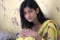Updated on 15-Jun-2020 15:20:04
Radix sort is a non-comparative sorting algorithm. This sorting algorithm works on the integer keys by grouping digits which share the same position and value. The radix is the base of a number system. As we know that in the decimal system the radix or base is 10. So for sorting some decimal numbers, we need 10 positional boxes to store numbers.The complexity of Radix Sort TechniqueTime Complexity: O(nk)Space Complexity: O(n+k)Input and OutputInput: The unsorted list: 802 630 20 745 52 300 612 932 78 187 Output: Data before Sorting: 802 630 20 745 52 300 612 932 78 187 ... Read More

## Quick SortUpdated on 15-Jun-2020 15:24:00
The quicksort technique is done by separating the list into two parts. Initially, a pivot element is chosen by partitioning algorithm. The left part of the pivot holds the smaller values than the pivot, and right part holds the larger value. After partitioning, each separate lists are partitioned using the same procedure.The complexity of Quicksort TechniqueTime Complexity: O(n log n) for best case and average case, O(n^2) for the worst case.Space Complexity: O(log n)Input and OutputInput: The unsorted list: 90 45 22 11 22 50 Output: Array before Sorting: 90 45 22 11 22 50 Array after Sorting: 11 22 ... Read More

## Pigeonhole SortUpdated on 15-Jun-2020 15:31:17
This is an example of the non-comparison sorting technique. It is used where the number of items and the range of possible key values is approximately the same.To perform this sort, we need to make some holes. The number of holes needed is decided by the range of numbers. In each hole, items are inserted. Finally deleted from the hole and stored into an array for sorted order.The complexity of Pigeon-Hole Sort TechniqueTime Complexity: O(n+2^k)Space Complexity: O(2^k)Input and OutputInput: The unsorted list: 802 630 20 745 52 300 612 932 78 187 Output: Data before Sorting: 802 630 20 745 ... Read More

## Merge Sort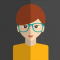Updated on 15-Jun-2020 15:27:06
The merge sort technique is based on divide and conquers technique. We divide the whole dataset into smaller parts and merge them into a larger piece in sorted order. It is also very effective for worst cases because this algorithm has lower time complexity for the worst case also.The complexity of Merge Sort TechniqueTime Complexity: O(n log n) for all casesSpace Complexity: O(n)Input and OutputInput: The unsorted list: 14 20 78 98 20 45 Output: Array before Sorting: 14 20 78 98 20 45 Array after Sorting: 14 20 20 45 78 98Algorithmmerge(array, left, middle, right)Input − The data set array, left, middle ... Read More

## Insertion SortUpdated on 15-Jun-2020 15:35:20
This sorting technique is similar with the card sorting technique, in other words, we sort cards using insertion sort mechanism. For this technique, we pick up one element from the data set and shift the data elements to make a place to insert back the picked up an element into the data set.The complexity of the Insertion Sort TechniqueTime Complexity: O(n) for best case, O(n^2) for average and worst caseSpace Complexity: O(1)Input and OutputInput: The unsorted list: 9 45 23 71 80 55 Output: Array before Sorting: 9 45 23 71 80 55 Array after Sorting: 9 23 45 55 ... Read More

## Heap Sort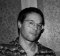Updated on 15-Jun-2020 15:39:25
Heap sort is performed on the heap data structure. We know that heap is a complete binary tree. Heap tree can be of two types. Min-heap or max heap. For min heap the root element is minimum and for max heap the root is maximum. After forming a heap, we can delete an element from the root and send the last element to the root. After these swapping procedure, we need to re-heap the whole array. By deleting elements from root we can sort the whole array.The complexity of Heap Sort TechniqueTime Complexity: O(n log n)Space Complexity: O(1)Input and OutputInput: A ... Read More

## Cycle SortUpdated on 15-Jun-2020 15:43:42
Cycle Sort is an in-place sorting algorithm. It is also a comparison based sort and efficient for any other in-place sorting technique. It finds the minimum number of memory write to perform the sorting tasks.The complexity of Cycle Sort TechniqueTime Complexity: O(n^2)Space Complexity: O(1)Input and OutputInput: A list of unsorted data: 23 63 98 74 20 14 36 45 99 78 Output: Array before Sorting: 23 63 98 74 20 14 36 45 99 78 Array after Sorting: 14 20 23 36 45 63 74 78 98 99AlgorithmcycleSort(array, size)Input − An array of data, and the total number in the ... Read More